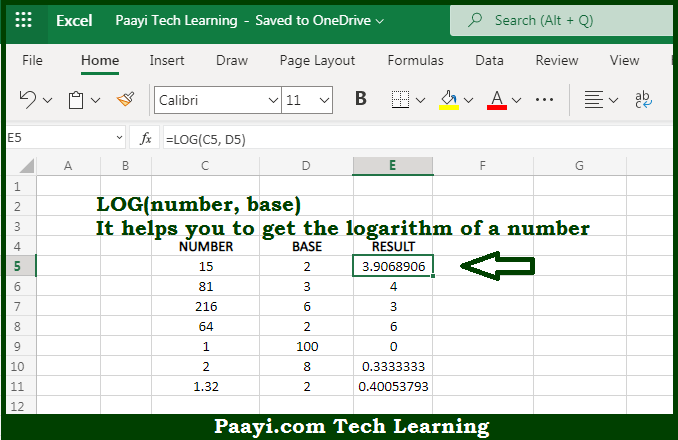# Learn How to Use Microsoft Excel LOG Function

Written by | 0 Comments | 626 Views

In this article, you will learn how to use the Microsoft Excel LOG function and its prime function in Microsoft Excel. You will also get to know the Microsoft Excel LOG function return value and syntax with the help of some examples.

Microsoft Excel LOG Function

The main function of the Microsoft Excel LOG function is to get the logarithm of the number. So, with the help of the LOG function, you can able to return the logarithm of a given number, using a base supplied. It should be noted that the base value defaults to 10, in case it is not provided. So, with the help of the LOG function, you can able to return the logarithm of the given number.

Return Value of LOG Function

The return value will be the logarithm.

Syntax of LOG Function

=LOG(number, [base])

Where the arguments:

• number: This is the number of which you want to get the natural logarithm.
• base: This is the base of the logarithm, that is defaults to 10 (optional).

How to Use Microsoft Excel LOG Function?So we know that Microsoft Excel LOG function you can able to return the logarithm of the given number. So, with the help of the LOG function, you can able to return the logarithm of a given number, using a base supplied. It should be noted that the base value defaults to 10, in case it is not provided. So, with the help of the LOG function, you can able to return the logarithm of the given number.